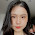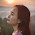## Thursday, February 3, 2011

### Duality for undergrads

The duality theorem of linear programming and its extensions is a fundamental pillar of optimization. It lies at the heart of an elegant theory which allows us not only to reason about the structure of continuous optimization problems, but also design combinatorial optimization and approximation algorithms.

John von Neumann said that In mathematics you don't understand things. You just get used to them. This is particularly true for duality (which von Neumann was the first to conjecture and essentially prove). I recall encountering linear programming duality as part of a graduate course. The standard pedagogy is first to try and write the dual program from the primal, and much later prove weak and strong duality, a very technical way indeed. All these years since, I still haven't gotten completely used to duality, but as close as I came is in teaching it this last semester, via a non-standard way,  to undergrads, many of whom have never taken a course in basic linear programming.

I'm familiar with three ways to prove duality. The first and hardest is via fixed-point theorems, as pioneered by von-Neumann in his proof of the minimax theorem. Don't try this on your undergrads.

The second, and most popular, is a geometric proof via the Farkas' lemma. This is usually grad-student material.

The way we tried this last semester proceeds as follows:

1. Define zero-sum games, and claim equivalence to linear programming. Zero sum games are clearly a special case, to show the other direction without resorting to heavy tools requires some care, as rigorously done by Adler. However, the intuition is very clear, and we did not go into details.

2. Explain the minimax theorem. Again - a very intuitive theorem, easier to grasp than duaity - and equivalent by (1).

3. Prove the minimax theorem on the board. We are not going to use toplogy (fixed-point theorems) nor geometry (Farkas), but an elementary construct called experts algorithms. The method of proving the minimax theorem via experts algorithms is due to Freund and Schapire.

The last step is of course the main one. Here's an almost precise account of the proof:  Recall that the minimax theorem for zero sum games tells us that

$$\lambda_A = \max_p \min_q p^T M q = \min_q \max_p p^T M q = \lambda_B$$

Here $M$ is the game payoff matrix (payoffs to the row player and costs to the column player), $p$ and $q$ are distributions over the strategies of the players. The relation $\max_p \min_q p^T M q \le \min_q \max_p p^T M q$ is called "weak duality", and follows easily from reasoning. The other direction, "strong duality", is where we need experts algorithms.

Consider a repeated game, in which the row player constructs $p_t$ at each iteration $t \in [T]$ according to an experts' algorithm. An experts algorithm guarantees the following: (this is the definition of an experts' algorithm)

$$\frac{1}{T} \sum_t p_t^T M q_t \geq \frac{1}{T} \max_{p_*} \sum_t p_*^T M q_t - o(1)$$

Here $t=1,2,...,T$ are the iterations of the repeated game, $p_t$ is the experts' mixed strategy at time $t$, and $q_t$ can be anything.

Define the average distributions to be: $\bar{p} = \frac{1}{T} \sum_t p_t$ and $\bar{q} = \frac{1}{T} \sum_t q_t$.

We have:

$$\lambda_A = \max_p \min_q p^T M q \geq \min_q \bar{p}^T M q = \min_q \frac{1}{T} \sum_t p_t^T M q$$

By minimizing over  q at each game iteration instead of minimizing over  q on all
game rounds, player B can only decrease his loss (and decrease player A's profit) and
hence:

$$\min_q \bar{p}^T M q \geq \frac{1}{T} \sum_t p_t M q_t$$

where $q_t = \arg \min_q p_t^T M q$.
By the low regret property we have

$$\frac{1}{T} \sum_t p_t^T M q_t \geq \max_p \frac{1}{T} \sum_t p^T M q_t - o(1)$$

Hence:
$$\frac{1}{T} \sum_t p_t M q_t \geq \max_p \frac{1}{T} \sum_t p M q_t - o(1) \geq \min_q \max_p p^T M q - o(1)$$

Overall we got:

$$\lambda_A \geq \lambda_B - o(1)$$
as needed.

1.Thanks for sharing such a nice article.I get good feelings everytime I read your posts.I think this article has a lot of information needed, looking forward to your new posts. Shopify free shipping bar app, App Shipping Bar Master, autoketing app

2.Thanks for sharing the post.Will come back again, Im taking your feed also,Love to read it,Waiting For More new Update and I Already Read your Recent Post its Great Thanks.
app autoketing, facebook inbox, facebook help chat

3.Somebody essentially help to make seriously articles I’d state. This is the very first time I frequented your website page and thus far? I amazed with the analysis you made to make this particular post amazing. Fantastic job!
autoketing.com
email with love by autoketing

4.I'm going to search and research my problem and see this web site, it really attracts me because a lot of useful information for everyone, I will often visit this site.
currency converter master, currency converter box by autoketing, best autoketing app

5.Your blog provided us with valuable information to work with. Each & every tips of your post are awesome. Thanks a lot for sharing. Keep blogging
iogames 2 player
abcya online games
friv land games

6.The story of cinderella Games to play
Crime city 3d 2 Games online
Top burger Games for girls
I'm a decent implied for all articles or blog entries, That I emphatically relished, We'd to a great degree pick much more records identified with the, because of the reality it is in reality okay

7.I hope you will write often and post more articles.I'm trying to learn more knowledge and your articles are so useful for me.They contain pieces of advice. I think many people love and need them. play Battle for the Galaxy Break The Cup For Free Game Toon Cup 2017

8.Delight maintain this approach awesome succeed and additionally Document wait for a great deal more on your stunning blog posts. I just want to let you know that I just check out your site and I find it very interesting and informative.
Ice cream maker game online
4 player sheep party best online game
Colorzzle online

9.Fire truck crazy race free
Free unblock the ball
Games princess and adult princess
Thanks for a very interesting blog. What else may I get that kind of info written in such a perfect approach? I’ve a undertaking that I am simply now operating on, and I have been at the look out for such info

10.Anyone who reads this post will find it great, me too, I will continue to follow your posts.
Cooking Christmas Traditional Food games online, Happy Glass free online, Hit The Glow for kids